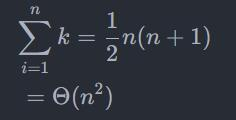# [Solved] Markdown Edit Formula Error: ParseError: KaTeX parse error: {align} can be used only in display mode

Problem description

Edit formula:\begin{align} & \sum\limits_{i=1}^{n}{k=\frac{1}{2}}n(n+1) \\ & \text{ }=\Theta ({{n}^{2}}) \\ \end{align}


Markdown Error: ParseError: KaTeX parse error: {align} can be used only in display mode
Error: KaTeX parse error: No such environment: align at position 7: \begin{̲a̲l̲i̲g̲n̲}̲ & \sum\limit…

Solution:
Modify {align} to {aligned}, KaTeX is not support align, you need to modify to aligned.
Layout
Next, modify the formula layout so that the formula surrounded by $$by KaTeX is centered. \limit can make n and i=1 located directly above and below the accumulation symbol. ​ $$
\begin{aligned}
\sum_{i=1}^{n} k &=\frac{1}{2} n(n+1) \\
&=\Theta \left(n^{2}\right)
\end{aligned}